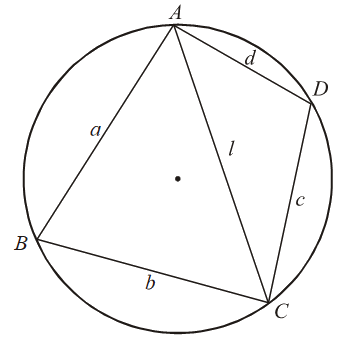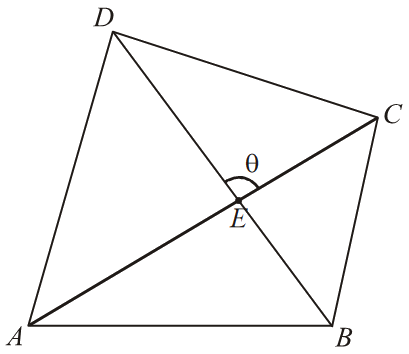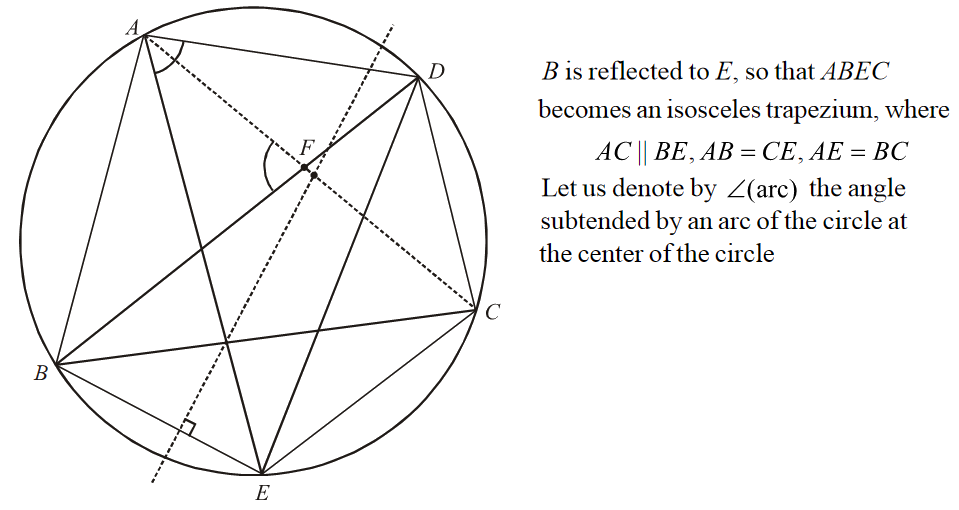# Trigonometry Set-3

Go back to  'SOLVED EXAMPLES'

Example - 7

Let $$ABCD$$ be a convex cyclic quadrilateral with sides $$a, b, c, d$$. If the semi-perimeter s of the quadrilateral is defined as \begin{align}s = \frac{{a + b + c + d}}{2}\end{align}, show that the area of the quadrilateral is

$\Delta = \sqrt {(s - a)(s - b)(s - c)(s - d)}$

Solution: Recall that a cyclic quadrilateral is one that can be inscribed in a circle, or in other words, whose vertices are concyclic.Using the cosine rule,

${l^2} = {a^2} + {b^2} - 2ab\cos B = {c^2} + {d^2} - 2cd\cos D \qquad \qquad \qquad ...(1)$

Since $$ABCD$$ is cyclic, \begin{align}\angle B + \angle D = \pi \end{align}, so that \begin{align}\cos D = - \cos B\end{align}.

Using this in (1), we have

\begin{align}\cos B = \frac{{{a^2} + {b^2} - {c^2} - {d^2}}}{{2(ab + cd)}} \qquad \qquad \qquad ...(2)\end{align}

The area of $$ABCD$$ can be expressed as the sum of areas of \begin{align}\Delta ABC\;{\text{and}}\;\Delta ACD\end{align}. Thus,

\begin{align}\Delta = \frac{1}{2}ab\sin B + \frac{1}{2}\;cd\;\sin D\end{align}

Since \begin{align}\angle B + \angle D = \pi \end{align}, we have

\begin{align}\Delta = \frac{1}{2}(ab + cd)\sin B\end{align}

From (2),

\begin{align} {\sin ^2}B & = 1 - {\cos ^2}B = (1 - \cos B)(1 + \cos B)\\ & = \frac{{\left[ {{{(a + b)}^2} - {{(c - d)}^2}} \right]\;\left[ {{{(c + d)}^2} - {{(a - b)}^2}} \right]}}{{4{{(ab + cd)}^2}}}\\ & = \frac{{(a + b + c - d)(a + b - c + d)(a - b + c + d)( - a + b + c + d)}}{{4{{(ab + cd)}^2}}}\\ & = \frac{{4(s - a)(s - b)(s - c)(s - d)}}{{{{(ab + cd)}^2}}}\\ \Rightarrow \qquad \Delta & = \sqrt {(s - a)(s - b)(s - c)(s - d)} \end{align}

Example - 8

$$AC$$ and $$BD$$ be \begin{align}\theta \end{align}. Let $$ABCD$$ be a quadrilateral, and let the angles between the diagonals.(a) Show that the area of $$ABCD$$ can be written as \begin{align}\Delta = \frac{1}{2}\;AC \cdot BD \cdot \sin \theta \end{align}

(b) If $$ABCD$$ is a convex cyclic quadrilateral, show that

\begin{align}AC \cdot BD = AB \cdot CD + AD \cdot BC\end{align}

Solution:(a) Note that

${\rm{area}}\;(\Delta ACD) = \frac{1}{2} \cdot AC \cdot ED\sin \theta$

and

${\rm{area}}\;(\Delta ABC) = \frac{1}{2} \cdot AC \cdot EB\sin \;(\pi - \theta ) = \frac{1}{2}.AC \cdot EB\sin \theta$

so that

\begin{align}\Delta = \frac{1}{2} \cdot AC \cdot (ED + EB) \cdot \sin \theta = \frac{1}{2}AC \cdot BD \cdot \sin \theta \end{align}

(b) We undertake a construction by reflecting $$B$$ along the perpendicular bisector of the diagonal $$AC.;$$Note that

\begin{align} \angle EAD & = \frac{1}{2}\;\angle (ED)\\ & = \frac{1}{2}\;\angle (EC + CD)\\ & = \frac{1}{2}\;\angle (AB + CD) & & {\rm{How?}}\\ & = \;\angle ADB + \angle CAD\\ & = \angle AFB = \theta \;({\rm{say}}) \end{align}

Make sure you are clear about how this result was obtained.

Also note by symmetry that area (ABCD) = area (AECD).

\begin{align} {\rm{area}}\;(ABCD) & = \frac{1}{2}AC \cdot BD \cdot \sin \theta \;\;(\text{by the result of part - (a))}\\ {\rm{area}}\;(AECD) & = {\rm{area}}\,(\Delta AED) + {\rm{area}}(\Delta ECD)\\ & = \frac{1}{2}AE \cdot AD \cdot \sin \theta + \frac{1}{2}EC \cdot CD \cdot \sin (\angle ECD)\\ {\rm{But}}\;\angle ECD = \pi - \theta \;{\text{due}}\;{\text{to}}&\;AECD\;{\text{being}}\;{\text{cyclic}}{\rm{.}}\;{\rm{Thus,}}\\ {\rm{area}}\;(AECD) & = \frac{1}{2}\sin \theta \;(AE \cdot AD + EC \cdot CD)\\ & = \frac{1}{2}\sin \theta (AD \cdot BC + AB \cdot CD)\;(\text{from remarks in}\;{\text{the}}\;{\text{figure}}) \end{align}

Comparing the two areas, the required equality is established.

Learn from the best math teachers and top your exams

• Live one on one classroom and doubt clearing
• Practice worksheets in and after class for conceptual clarity
• Personalized curriculum to keep up with school